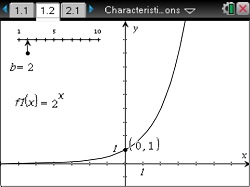Characteristics of Exponential Functions

Investigate how the graph of an exponential function changes when 0 < b < 1, b = 1, or b > 1.
•TI-Nspire™ CX/CX II
•TI-Nspire™ CX CAS/CX II CAS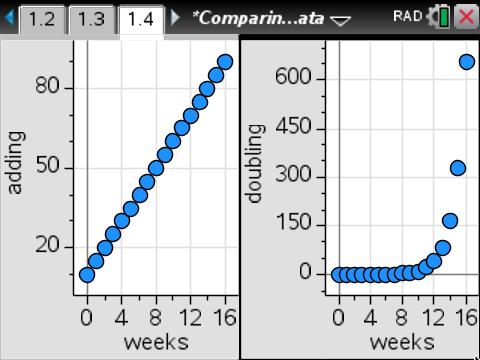Comparing Linear and Exponential Data

Compare a linear and an exponential relationship.
•TI-Nspire™ CX/CX II
•TI-Nspire™ CX CAS/CX II CAS
• TI-Nspire™
• TI-Nspire™ CAS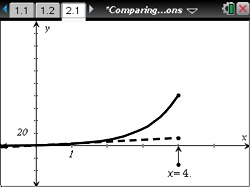Comparing Linear and Exponential Functions

Compare data from two different scenarios -- linear and exponential growth.
•TI-Nspire™ CX/CX II
•TI-Nspire™ CX CAS/CX II CAS
• TI-Nspire™
• TI-Nspire™ CASComparing Linear and Exponential Functions

Compare data from two different scenarios -- linear and exponential growth.
•TI-Nspire™ CX/CX II
•TI-Nspire™ CX CAS/CX II CAS
• TI-Nspire™ Navigator™
• TI-Nspire™
• TI-Nspire™ CAS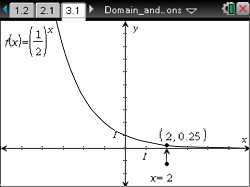Domain and Range of Exponential Functions

Determine the domain and range of an exponential function f(x) = bx.
•TI-Nspire™ CX/CX II
•TI-Nspire™ CX CAS/CX II CAS
• TI-Nspire™ Navigator™
• TI-Nspire™
• TI-Nspire™ CAS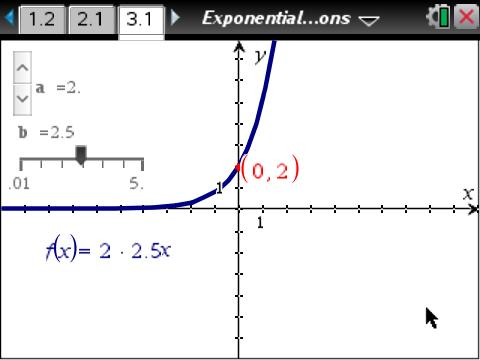Exponential Functions f(x) = ab<sup>x</sup>

Move a slider to investigate the effect of different a-values on f(x) = a*bx.
•TI-Nspire™ CX/CX II
•TI-Nspire™ CX CAS/CX II CAS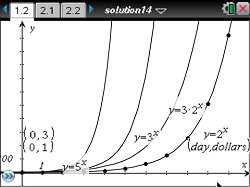Exponential Growth

Model exponential growth and graph equations of the form y = a*bx.
•TI-Nspire™ CX/CX II
•TI-Nspire™ CX CAS/CX II CAS
• TI-Nspire™
• TI-Nspire™ CAS••